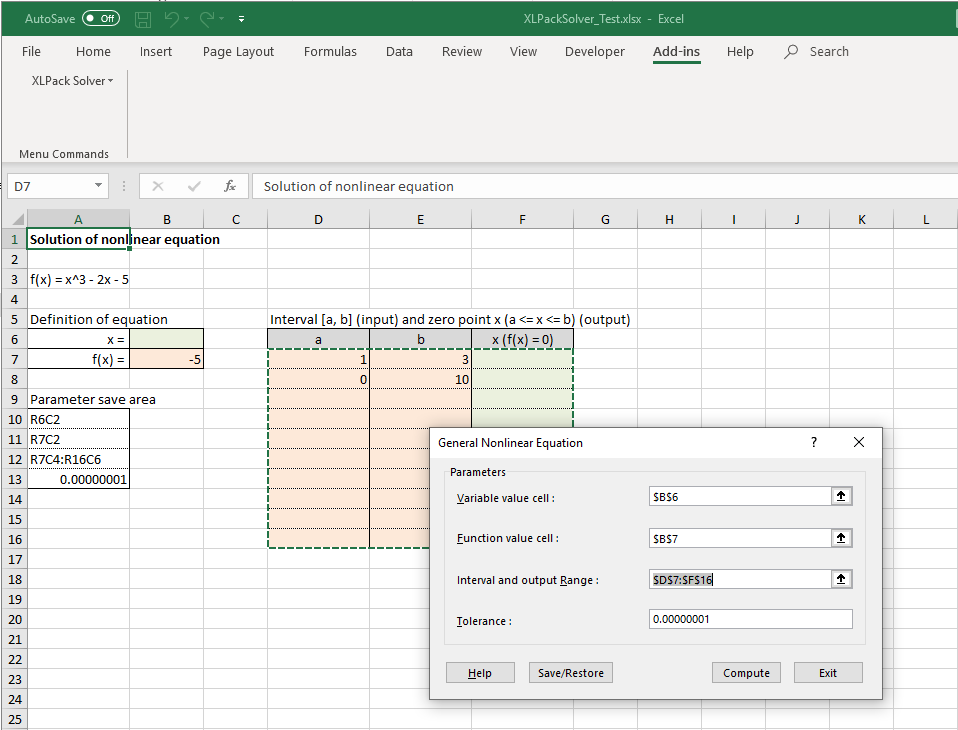# XLPack Solver – General Nonlinear Equation

Solve the general nonlinear equation with single variable using Dfzero.

Let’s solve the following example equation.

f(x) = x^3 – 2x – 5 = 0

The solver program will write the value of x into the variable value cell (B6 in this case). The function value cell (B7 in this case) must contain the formula to compute f(x) from x. To solve this example, the formula is =B6^3-2*B6-5.

The interval and output range (D7:F8 in this case) is the cell range for search interval [a, b] (input) and the zero-point x (output). Input a and b in the first and second columns of each row and click “Compute”. Then the value of x between a and b satisfying f(x) = 0 will be computed and output to the third column of each line. If the row with a blank cell in the first or second column is encountered, the computation will be terminated even within the interval and output range.

The standard value of tolerance is 1.0e-8. The tolerance value will be set to Re and Ae of Dfzero.

The cell ranges can be specified as larger than required. In that case, only the necessary range will be used from left upper corner.Please refer to here for “Save/Restore” button.

When “Help” button is clicked, this page will be displayed if the network connection is available.

The “?” button at the right upper corner will not work correctly. Please use “Help” button.